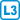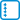## Prescribed edge in cubic graph

Let $$G$$ be a cubic graph without bridges. Prove that for each of its edges there is a perfect matching that contains it.

• #### Hint

Similarly to the task where the matching does not contain a specified edge. The graph must have an even number of vertices. Remove both vertices that form the ends of the edge.

• #### Solution

Let’s denote the graph by $$G$$ and the prescribed edge by $$uv$$. By $$G’$$ we denote the graph $$G$$ after removing the vertices $$u, v$$.

For a contradiction, suppose that $$G’$$ does not have a perfect matcing and therefore Tutte’s condition does not hold. Hence there exists $$S’\subseteq V (G’)$$ that $$| S’ | < C_o (G’, S’)$$.

Cubic graphs such as $$G$$ have an even number of vertices according to the principle of evenness, and the same is true for $$G’$$. As a consequence, the size of the $$S’$$ and the number of odd components have the same parity. Therefore, the inequality $$| S’| < C_o (G’, S’)$$ can be strengthened to $$| S’| \le C_o (G’, S’) - 2$$.

To obtain the cut $$S$$ in the graph $$G$$, we choose $$S = S’\cup \{u, v \}$$. The complement of $$S$$ in $$G$$ is the same as the complement of $$S’$$ in $$G'$$, therefore $$C_o (G, S) = C_o (G’, S’)$$.

According to the principle of evenness, an odd number of edges should lead to the odd components of the graph $$G$$. Because the graph $$G$$ has no bridge, at least three edges connect each odd component.

Because $$G$$ is cubic, from $$S’$$ stems at most $$3|S’|$$ edges. After adding the vertices $$u$$ and $$v$$, we get that from $$S$$ stems at most $$3 |S’|+4$$ edges.

Therefore, for the number of edges $$\#e$$ incident with $$S$$ we get that: $3 (C_o (G, S)) \le \#e \le 3 | S’| +4 \le 3 (C_o (G’, S’) - 2) +4 = 3 (C_o (G, S)) -2$ which is the desired contradiction.